﻿ Using Artificial Neural Network to Predicted Student Satisfaction in E-learningPublications are Open
Access in this journal
Article Versions
Export Article
• Normal Style
• MLA Style
• APA Style
• Chicago Style
Open Access Peer-reviewed

### Using Artificial Neural Network to Predicted Student Satisfaction in E-learning

Dalia Kamal Fathi Alnagar
American Journal of Applied Mathematics and Statistics. 2020, 8(3), 90-95. DOI: 10.12691/ajams-8-3-2
Received August 04, 2020; Revised September 05, 2020; Accepted September 14, 2020

### Abstract

In this study, a constructed multi-layer perceptron artificial neural network model was created.. This study examines the determinants of student satisfaction in E-learning and proposes a model for identifying factors that influence student satisfaction using artificial neural networks for students at Tabuk University. The study model conducting using a questionnaire survey of 321 participants who studied E-learning, attitudes, and reactions of instructors, the flexibility of E-learning courses, interaction in virtual classrooms, and E-learning according to various evaluations. It is carried out using a prediction of student satisfaction. The workshop and prepared explanations helped students in the use of E-learning, the quality of the internet, and the nature of the course E-learning in the Dean's office. The model predicted student satisfaction with E-learning per 92.2% correct classification rate (CCR). The values in the region below the ROC curve (AUC) of the model were rated excellent (0.990%). The results show that different assessments are a powerful determinant of learning satisfaction.

### 1. Introduction

E-learning is a learning style supported by digital technology and generally refers to learning over the Internet. Recently, E-learning has become an important fact and has been accepted by various institutions as a promising solution for providing students with on-demand learning opportunities to improve their learning process. The concept of E-learning is understood in many aspects, including flexible Internet environments, distributed computing, virtual learning environments, and general distance learning 1.

Many factors such as structure, quality of support systems, quality of content, quality of support networks, evaluation, and diversity of peer support networks can affect the E-learning experience 2, 3.

Therefore, universities and teachers need to consider these factors to help students learn well. Therefore, it is important to investigate the determinants of student satisfaction in E-learning 4, 5.

Student satisfaction is an important measure of the effective implementation and accomplishment of E-learning.

Overall, satisfaction is a factor used to monitor the quality of a product or service and predict the outcome of customer satisfaction or other behavior. This study focuses on factors related to university or faculty management that have influenced student satisfaction 6. In this study, we will create a model that can predict student satisfaction using an artificial neural network. Independent variables include instructor attitudes and responses in virtual classrooms, E-learning course flexibility, course types, virtual interactions, various assessments, internet quality, and technology quality. Student satisfaction is discussed as a dependent variable. Seven independent variables are described and supported in the literature to test each variable associated with the dependent variable. They have been studied as inputs for building artificial neural network (ANN) models that can predict student satisfaction from the student's point of view.

### 2. Goals and Hypothesis

The goal of this study is to create an artificial neural network (ANN) model that can predict student satisfaction with E-learning in order to investigate the factors that influence student satisfaction and raise student satisfaction. It was that. Student satisfaction is discussed as a dependent variable. A total of seven hypotheses have been proposed and supported by the literature to test the relationship of each variable to the dependent variable.

Hypothesis 1: Instructor Attitude and Response

will significantly affect student satisfaction with E-learning.

Previous study has shown that instructor attitude and responsiveness have a favorable impact on student satisfaction with E-learning 1.

Hypothesis 2: flexibility

The flexibility of the ELearning courses will improve student satisfaction with E-learning.

Hypothesis 3. Interaction in a virtual class

Interaction in a virtual class will increase student satisfaction with E-learning.

Many experts think that interactive instructional design is an important aspect in determining learner satisfaction and success 1.

Hypothesis 4. Workshop and explanations are Introduced

Workshop and explanations are introduced.

The workshop and explanations given will have a beneficial impact on student satisfaction with E-learning.

Workshops and explanations are provided to assist students in using technology.

Hypothesis 5. Diversity in Assessments

Diversity in Assessments will increase student satisfaction with E-learning.

The use of various assessment methods in E-learning provides students with pleasure in their achievement and ensures that their learning efforts are appropriately measured 1.

Internet Speed

Hypothesis 6.Internet speed

The quality of the internet will have a favorable impact on student satisfaction with E-learning. Internet speed enables students to participate in virtual class lectures and interactions.

E-learning Course Type and quality

Hypothesis 7. The sort of E-learning course will have a positive effect on student satisfaction with E-learning.

The kind and quality of course content is a critical factor in student satisfaction.

### 3. Materials and Methods

The human brain can be formed by a collection of artificial neurons using machine learning methods defined as neural networks 7.

Artificial neural networks (ANNs) are computational systems that may solve complex problems by basically mimicking human brain functions 8. Artificial neurons or nodes, which are information processing units that are stacked and connected by synaptic weights, are used in perceptron-type neural networks (connections).

Neurons may filter and transfer information in a controlled manner in order to construct prediction models that categorize data stored in shared memory.

The ANN model is composed of three layers of linked nodes:

- the input layer

- the hidden layer,

- the output layer.

One or more hidden layers may be formed by the nodes that connect the input and output levels. The input layer gathers information from the outside world, the hidden layer analyzes it, and the output layer provides class labels or anticipates continuous values. The value from the input layer is multiplied by a collection of integers, the weight, and the product is added to generate a single number. This number is fed into the activation function, a nonlinear mathematical function that returns a value between zero and one. A neural network is seen in Figure 1.

The following components make up artificial neural networks 7.

The net sum of the weighted input entering node j and the output activation function (most commonly used is the sigmoid function) that transforms the weighted input of a neuron into its activation output is given by: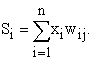(1)

And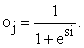(2)

Neurons and ANNs have two modes of operation: training mode and use mode. The training process teaches the algorithm how to anticipate the outcome using actual input and output information. This supervised learning starts with random weights and modifies them to the task at hand utilizing gradient descent search techniques like backpropagation. In the error function, the difference between the planned output value and the learnt value is used to drive learning 9. The weights that must be changed in order to minimize the error determine the error function. For a given training set (x1, t1), (x2, t2),..., (xk, tk), there are n inputs and m dimension vectors (n-inputs, m-outputs). It is made up of ordered pairs. The output pattern is shown, and the output error for each neuron may be calculated using the following equation: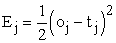(3)

The error function of the minimized network is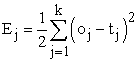(4)

where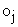is the output produced when the input pattern is used.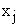from the training set is introduced into the network, and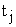is the goal value 9. During training mode, each weight is modified by adding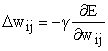(5)

Here, γ is a constant that indicates the learning rate. The faster the convergence, the higher the learning rate, yet the search route might become trapped in the best answer, making it hard to converge. Once the suitable set of weights is established, the neural network model may obtain another dataset with an unknown output value and forecast it automatically 12.

Artificial neural networks (ANNs) are among the most powerful modeling applications that have been used in statistical modeling 13.

### 4. Data Description

The data for this study was collected at the University of Tabuk in Ksa during the second semester of the 2019-2020 academic year. 321 students from the faculty of science were chosen at random and studied in the Blackboard system.

Blackboard is a virtual learning environment and learning management system that maintains records, reports, or any achievements of various user actions.

This study's questionnaire items were based on previous research. The ANN is built using seven variables. For the weight, a 5 Likert scale ranging from 1 as strongly disagree to 5 as strongly agree was employed. E-learning Course flexibility.

1. Instructor attitude and response

2. Interaction in virtual classroom

3. Diversity in assessment

4. Workshop and explanations introduced (Technology quality)

5. Internet speed

6. Type of learning course

Dependent variable:

As a binary dependent variable, student satisfaction has two values: 0 and 1. The number 0 indicates that the dependent variables are unsatisfied, whereas the value 1 indicates that the student is satisfied.

### 5. ANN Design and Setup

MLPs are generally multilayer feed forward networks that have been trained with static backward propagation. These networks have made their way into a wide range of applications requiring static pattern categorization 15. We built a neural network model and tested its accuracy using the IBM SPSS Statistics 25 (MLP) module. An MLP neural network trained with a back propagation learning algorithm that uses the steepest descent approach to update weights and minimizes error functions. Data was randomly assigned to a subset of training (60%), tests (20%), and holdouts (10%). (20 percent). Weights and models are built using training datasets. During training mode, the test data is utilized to detect faults and prevent overtraining. The model is validated using holdout data 10. The softmax function accepts real values as parameters and converts them to real numbers with sums ranging from 0 to 1. Second, because the total of the output activations is 1, the softmax layer may be thought of as a probability distribution, and the 0 j value can be described as the network's estimated likelihood of categorizing the input. 12

### 6. Result

This study was conducted to help universities increase their satisfaction by predicting student satisfaction in E-learning (satisfaction or dissatisfaction) and creating MLP neural network models that can analyze the data. Table 1 provides information about the dataset used to create the ANN model. Table 1 shows that there are 191 training samples, 66 test samples, and 64 holdout samples. A total of 321 cases.

• Figure 1. Network diagram

Figure 1 shows a network diagram used to predict student satisfaction (unsatisfaction = 0, satisfaction = 1) using seven input variables, as shown in Figure 1. The graph shows 7 input nodes, 4 hidden nodes, and 2 output nodes, showing the satisfaction and dissatisfaction categories.

According to the model summary table, the proportion of wrong predictions based on training and testing samples is 4.7 percent and 4.5 percent, respectively, whereas the rate of inaccurate predictions in the holdout data set is 7.8 percent. The learning technique was repeated until 10 consecutive steps with no drop in error function were obtained from the testing sample.

The expected student satisfaction E-learning is characterized as fulfilled in each case if the predicted probability is greater than 0.5. As can be observed, the MLP network accurately identified 182 students in the training sample and 63 students in the testing sample out of 191.

Overall, 95.6 percent of the training instances were categorized correctly.

In the holdout sample, the sensitivity was 88.2 percent, the specificity was 93.6 percent, and the model's accuracy was 92.2 percent, indicating a very accurate model.

The MLP network model incorrectly identified 5 students (7.8%) as false positives. The chance of predicting satisfaction for a student who would be dissatisfied should be as low as possible.

Figure 2 depicts the ROC diagram, which demonstrates that the classification performance for all possible thresholds is correct. The area value under AUC is 0.990, which indicates that the model-predicted pseudo-probability for the student to be in the satisfied category is outstanding.

Figure 3 depicts the relative and normalized relevance of the variables, as well as the influence of each independent variable in the ANN model. The chart depicts; the normalized importance of a diversity of assessments ratio is highest whereas instructor ratio has least normalized importance.

• Figure 3. Neutral network importance chart

The results show that the different types of exams and E-learning courses have a significant impact on the satisfaction of students.

Table 4 shows that the diversity of assessments were the most important determinant of student's satisfaction in E-learning.

### 7. Conclusion

This study aimed to construct the effectiveness of artificial neural networks in predicting student satisfaction, based on data collected from students' E-learning. The literature background indicated that neural networks exceed all other classifiers, in terms of accurate prediction. A multilayer perceptron neural network was published by the back-propagation algorithm, to predict students' satisfaction in E-learning.

The classification accuracy rate was high, with 92.2% accurate in classifying the students into the predicted satisfied and unsatisfied categories. The empirical results indicate that diversity in assessments and type of E-learning course learning are two strong determinants of learning satisfaction. The results from the study also show an AUC is 0.990 has excellent discrimination ability. The results of this study are very interesting, drawing attention to them, inviting the researcher, and encouraging them to conduct similar future studies that expand and deepen a study student satisfaction with E-learning.

Regardless of future studies will need to investigate these results in a larger area and diverse samples, there are positive results that the proposed model can be able to predict student satisfaction and help the university to raise student satisfaction in E-learning.

### Acknowledgements

Students of Faculty science, University of Tabuk was strongly acknowledged for participating in the questionnaire for research.

### References

  Stefanovic, Darko, et al. “Empirical study of student satisfaction in E-learning system environment.” Technics technologies education management 6.4 (2011), 1152-1164. In article  Arbaugh, J. B. Virtual classroom characteristics and student satisfaction with internet based MBA courses. Journal of Management Education, 24(1), (2000). 32-54. In article  Areti, V. Satisfying distance education students of the Hellenic Open University. E-mentor, 2 (14), (2006). 1-12. In article  Bender, D. M., Wood, B. J., & Vredevoogd, J. D. Teaching time: Distance education versus classroom instruction. The American Journal of Distance Education, 18 (2), (2004), 103-114. In article  Roberts, T. G., Irani, T. A., Telg, R. W., & Lundy, L. K. The development of an instrument to evaluate distance education courses using student attitudes. The American Journal of Distance Education, 19 (1), (2005): 51-64. In article  Fullerton, G., Taylor, S. “Mediating, interactive, and non-linear effects in service quality and satisfaction with services research”, Canadian Journal of Administrative Sciences, Vol. 19 No.2, (2002), pp.124-36. In article  Eswari, J. Satya, et al. “Prediction of stenosis behaviour in artery by neural network and multiple linear regressions.” Biomechanics and Modeling in Mechanobiology. (2020): 1-15. In article  McClelland, J.L., Rumelhart, D.E., and Hinton, G.E. The appeal of parallel distributed processing, in Parallel Distributed Processing: Explorations in the Microstructure of Cognition - Foundations, Vol.1, MIT Press, Cambridge, (1986), pp.3-44. In article  Leverington.. A Basic Introduction to Feedforward Backpropagation Neural Networks In article  http://www.webpages.ttu.edu/dleverin/neural_network/neural_networks.htm, (2009). In article  Rojas Raúl. Neural Networks: A Systematic Introduction, Springer-Verlag, Berlin, New-York. ,(1996) In article  IBM .Knowledge Center. http://goo.gl/SuuMHu, (2016). In article  R.Ruhin kouser, J.Daphney Joann, K. Suganya, FORECASTING STUDENT ACADEMIC PERFORMANCE BY DECISION TREE LEARNING USING ARTIFICIAL NEURAL NETWORKS, International Research Journal of Engineering and Technology (IRJET) e-ISSN: 2395 -0056 Volume: 03 Issue: 12 | Dec -2016. In article  Zacharis, Nick Z. “Predicting student academic performance in blended learning using Artificial Neural Networks.” International Journal of Artificial Intelligence and Applications 7.5 (2016): 17-214. In article  Tufaner, Fatih, and Yavuz Demirci. “Prediction of biogas production rate from anaerobic hybrid reactor by artificial neural network and nonlinear regressions models.” Clean Technologies and Environmental Policy (2020): 1-12. In article  Asogwa, O. C., et al. “On the Modeling of the Effects of COVID-19 Outbreak on the Welfare of Nigerian Citizens, Using Network Model.” American Journal of Applied Mathematics and Statistics 8.2 (2020): 58-63. In article  asser, Ibrahim M., and Samy S. Abu-Naser. “Predicting Tumor Category Using Artificial Neural Networks.” (2019). In article  O. C. Asogwa and A. V. Oladugba .Of Students Academic Performance Rates Using Artificial Neural Networks (ANNs). American Journal of Applied Mathematics and Statistics.3, (4) (2015): 151-155. In article  Dziuban, Charles, et al. “Student Satisfaction with Online Learning: Is It a Psychological Contract?.” Online Learning 19.2 (2015): n2. In article  Wu, Jen-Her, Robert D. Tennyson, and Tzyh-Lih Hsia. “A study of student satisfaction in a blended E-learning system environment.” Computers & Education 55.1 (2010): 155-164. In articleThis work is licensed under a Creative Commons Attribution 4.0 International License. To view a copy of this license, visit http://creativecommons.org/licenses/by/4.0/